## Summary

For many effects we want random numbers to generate patterns or other things in our shaders. Directly using those random values generates a pattern we call “white noise”. There are other patterns which have more structure we can generate based on that which we will explore in other tutorials, for example perlin and voronoi noise. For this tutorial we will implement the noise in a surface shader so you should know how to write a basic surface shader).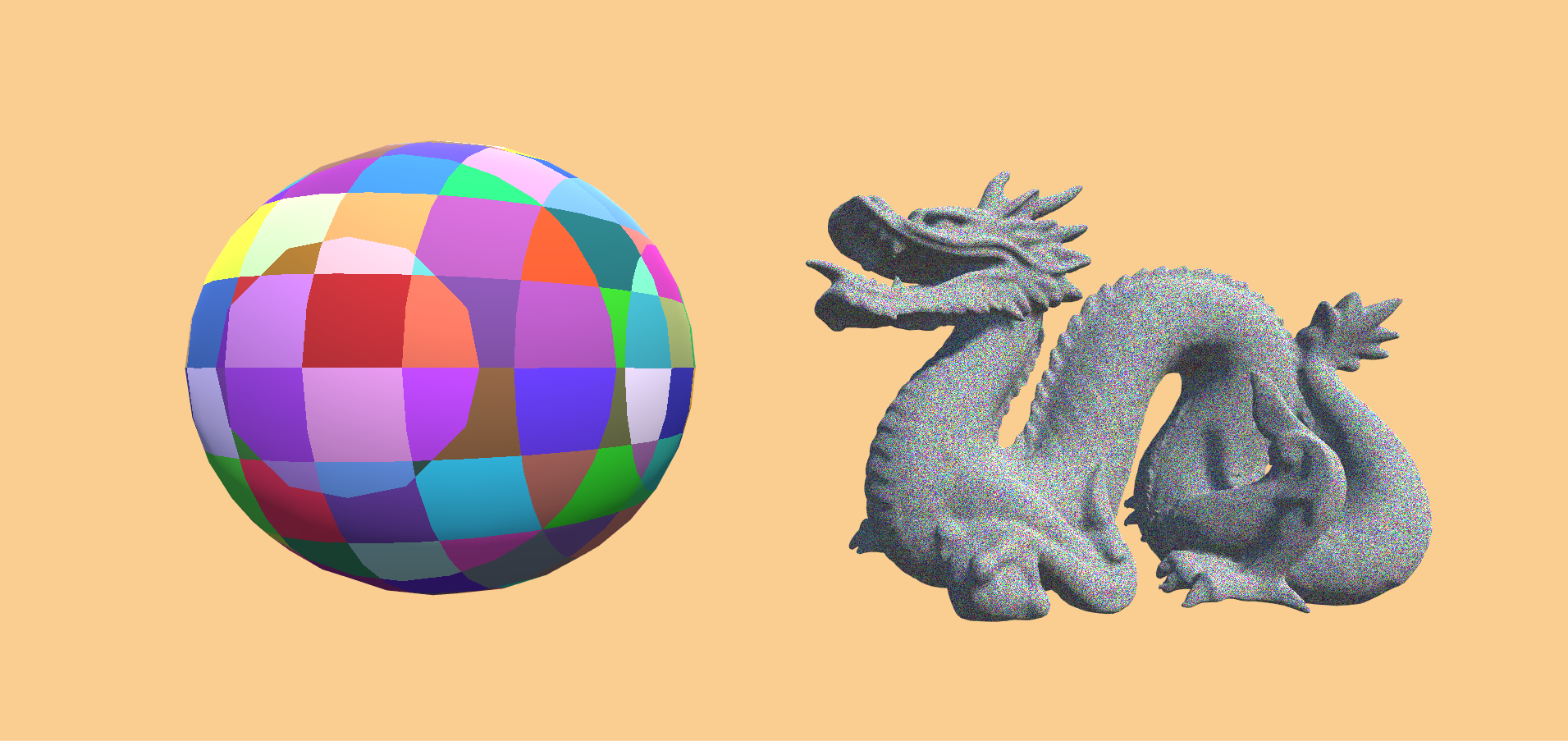## Scalar noise from 3d Input

In shaders we can’t easily save variables from one frame of rendering to the next, so our random numbers have to depend on the variables we do have access to. For this example I’m going to use the world position, but if we want animated noise, we can also factor in the time.

To have access to the world position we just have to add a variabel called `worldPos` to our input struct. We’ll also remove the uv coordinates for the texture because we won’t use it for the example.

``````struct Input {
float3 worldPos;
};
``````

Then we’ll write the random generation of the noise values in their own function to make it easy to use it at different positions and reuse it in other shaders. The first iteration of the function will take any 3d value and return a random scalar value with components between 0 and 1. the first step is to convert the 3d value we have into a scalar 1d value. A easy way that works for our purposes here is to take the dot product with another 3d vector. The resulting value will become really high though, so we take the result of the dot product and only take the fractional part, the part after the `.` of a decimal number. We can do that in hlsl with the `frac` function.

``````float rand(float3 vec){
float random = dot(vec, float3(12.9898, 78.233, 37.719));
random = frac(random);
return random;
}
``````

If we now call the function with the world position as a parameter and write the result to the albedo of the material we can already see a result.

``````void surf (Input i, inout SurfaceOutputStandard o) {
o.Albedo = rand(i.worldPos);
}
``````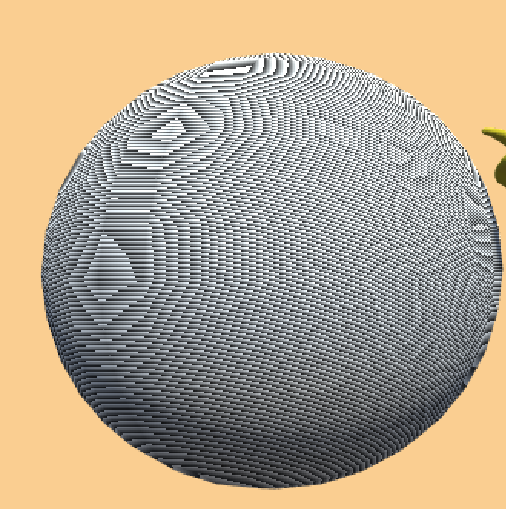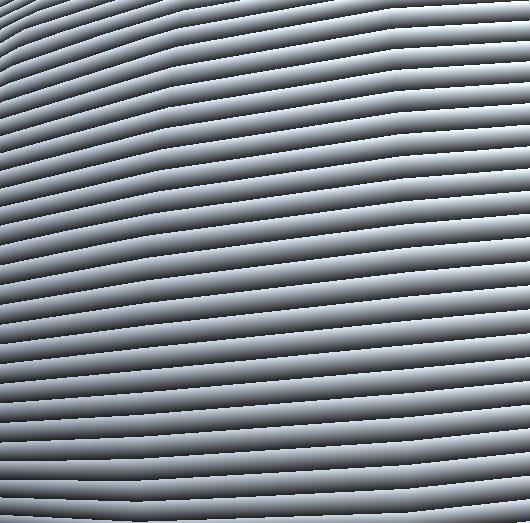The biggest problem with the values we get from the function is that we can see fairly quickly that they’re not “very random”, we can see the bands the dot function creates. The solution to this might seem hacky, but it’s fast and works for our cases. We simply multiply the random value with a very high number before taking the fraction so the bands become so small we can’t see them anymore.

``````float rand(float3 vec){
float random = dot(vec, float3(12.9898, 78.233, 37.719));
random = frac(random * 143758.5453);
return random;
}
``````Theres a new problem that arises from that though. Namely that by multiplying the value by a high number, we quickly move towards the maximum range that floating point numbers can represent when we move our object away from the origin of the scene.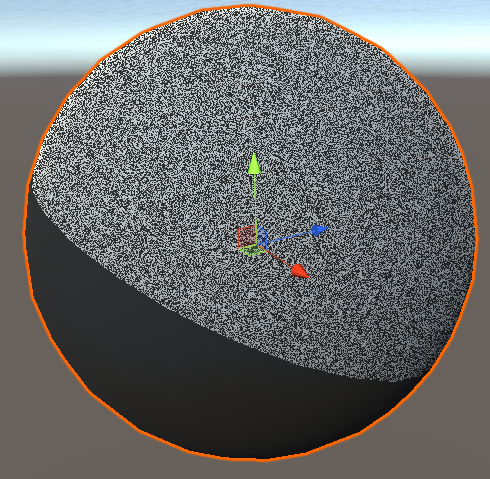The fix for that is to use a operation that limits the range of the random value to a low number before multiplying it with a big number. I just use a sine function here (apparently they’re just a expensive as multiplications or additions in shaders because they’re calculated in special calculation units, who knew…). And if we’re concerned about artafects when looking really close at the material when the object has a position in the low thousands we can also use a sine function to limit the range of the input vector before taking the dot product.

``````//get a scalar random value from a 3d value
float rand(float3 value){
//make value smaller to avoid artefacts
float3 smallValue = sin(value);
//get scalar value from 3d vector
float random = dot(smallValue, float3(12.9898, 78.233, 37.719));
//make value more random by making it bigger and then taking teh factional part
random = frac(sin(random) * 143758.5453);
return random;
}
``````

## Different Input and Output

To output more dimensional vectors we can simply call the function multiple times for different directions, but we have to use different parameters so we don’t get the same value for all axes. The easiest way to do that is to take the dot product with a different vector. To pass a different vector to the rand function we allow the caller to pass a different value to take the dot product with, but also allow the possibility to not do that and use the previous value as a default value. Because we add more random functions, we will also rename the existing one to `rand3dTo1d` to make the difference to the other ones clear.

``````//get a scalar random value from a 3d value
float rand3dTo1d(float3 value, float3 dotDir = float3(12.9898, 78.233, 37.719)){
//make value smaller to avoid artefacts
float3 smallValue = sin(value);
//get scalar value from 3d vector
float random = dot(smallValue, dotDir);
//make value more random by making it bigger and then taking teh factional part
random = frac(sin(random) * 143758.5453);
return random;
}
``````

To write a 3d to 3d noise function we can now call the 3d to 1d one 3 times, once per component of the random vector we’re returning, each time with a different vector to do the dot product with. This will then create more colorful noise, in opposition to the greyscale noise we had previously. The reason we call the existing function three times instead of writing a new one and not converting the 3d value to a scalar value is that this way we make sure the different components are independent from each other, otherwise the x output would be mainly driven by the x input etc. which can lead to undesirable results.

``````//get a 3d random value from a 3d value
float3 rand3dTo3d(float3 value){
return float3(
rand3dTo1d(value, float3(12.989, 78.233, 37.719)),
rand3dTo1d(value, float3(39.346, 11.135, 83.155)),
rand3dTo1d(value, float3(73.156, 52.235, 09.151))
);
}
``````
``````void surf (Input i, inout SurfaceOutputStandard o) {
o.Albedo = rand3dTo3d(i.worldPos);
}
``````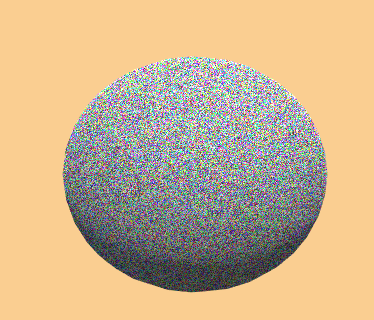To create functions that take a 2d input we simply take the dot product with another 2d vector to get a scalar value. And to use a scalar input we just leave out the step with the dot product because we don’t need to convert it to a scalar value, as a variable to get different results for the same input, we’ll add a mutator variable similar to the vector for the multiple dimension input methods. We then add the mutator to our input variable before doing the other random operations. Having a default mutator that’s not 1 and is added, also has the advantage that when we input a value of 0 into the function we don’t get 0 as a result, which could lead to weird artefacts otherwise. With this knowledge we can create 9 methods that take all different inputs and outputs. The advantage of writing all of them down now, is that we never have to write them down again. We don’t even have to copy them if we put them in a include file.

``````//to 1d functions

//get a scalar random value from a 3d value
float rand3dTo1d(float3 value, float3 dotDir = float3(12.9898, 78.233, 37.719)){
//make value smaller to avoid artefacts
float3 smallValue = sin(value);
//get scalar value from 3d vector
float random = dot(smallValue, dotDir);
//make value more random by making it bigger and then taking the factional part
random = frac(sin(random) * 143758.5453);
return random;
}

float rand2dTo1d(float2 value, float2 dotDir = float2(12.9898, 78.233)){
float2 smallValue = sin(value);
float random = dot(smallValue, dotDir);
random = frac(sin(random) * 143758.5453);
return random;
}

float rand1dTo1d(float3 value, float mutator = 0.546){
float random = frac(sin(value + mutator) * 143758.5453);
return random;
}

//to 2d functions

float2 rand3dTo2d(float3 value){
return float2(
rand3dTo1d(value, float3(12.989, 78.233, 37.719)),
rand3dTo1d(value, float3(39.346, 11.135, 83.155))
);
}

float2 rand2dTo2d(float2 value){
return float2(
rand2dTo1d(value, float2(12.989, 78.233)),
rand2dTo1d(value, float2(39.346, 11.135))
);
}

float2 rand1dTo2d(float value){
return float2(
rand2dTo1d(value, 3.9812),
rand2dTo1d(value, 7.1536)
);
}

//to 3d functions

float3 rand3dTo3d(float3 value){
return float3(
rand3dTo1d(value, float3(12.989, 78.233, 37.719)),
rand3dTo1d(value, float3(39.346, 11.135, 83.155)),
rand3dTo1d(value, float3(73.156, 52.235, 09.151))
);
}

float3 rand2dTo3d(float2 value){
return float3(
rand2dTo1d(value, float2(12.989, 78.233)),
rand2dTo1d(value, float2(39.346, 11.135)),
rand2dTo1d(value, float2(73.156, 52.235))
);
}

float3 rand1dTo3d(float value){
return float3(
rand1dTo1d(value, 3.9812),
rand1dTo1d(value, 7.1536),
rand1dTo1d(value, 5.7241)
);
}
``````

To put the functions in a include file we create a new file in our project and call it `WhiteNoise.cginc`. We have to change the name manually outside of Unity because it doesn’t recognize the file ending. Then we copy all of our noise functions into the new file. Then in the original shader we just add the line

``````#include "WhiteNoise.cginc"
``````

somewhere at the top of our cgprogram and we have access to all of the functions without having them cluttering our main file.

To make sure we don’t include our file multiple times in the future we can add a “include guard” around the functions in the file. If we don’t do that and somehow accidentally include the file twice the compiler will complain about multiple functions having the same name even though it’s the same function multiple times.

The include guard first checks if the file was not already included via `#ifndef WHITE_NOISE` then, if it hasn’t, we can declare it as included with the line `#define WHITE_NOISE` and at the end of the file we can end the part that’s only used when white noise isn’t define with `#endif`.

``````#ifndef WHITE_NOISE
#define WHITE_NOISE

//our library functions

#endif
``````

## Cells

Right now we generate the random positions based on the exact position of the surface we render. Because those values are so exact and small, the result looks very noisy(duh’) and changes a lot as soon as we move the object or the camera. A solution for that is to divide space into separate cells and generate the same random value for every point in a cell. The only thing we need to do to put our points into cells is to get the same value for all points in a cell. We do this by flooring the input, so for example all values from 1.0 to 2.0 will all use 1.0 as a value to generate a color.

``````void surf (Input i, inout SurfaceOutputStandard o) {
float3 value = floor(i.worldPos);
o.Albedo = rand3dTo3d(value);
}
``````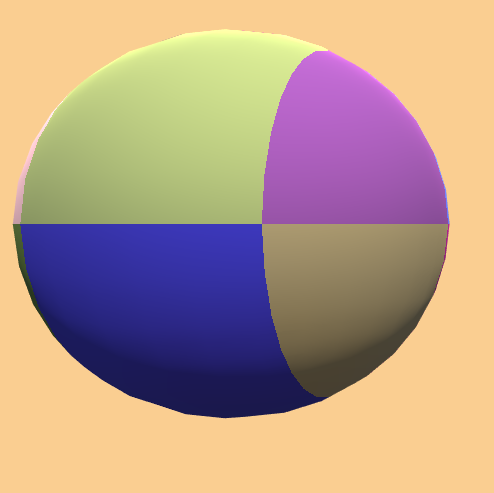Now that we have clear cells which have distinct values, next we want to change the size of those cells. We do this with a new property.

``````Properties {
_CellSize ("Cell Size", Vector) = (1,1,1,0)
}
``````
``````float3 _CellSize;
``````

Now that we have all variables we need, we simply divide the world position by the cell size before we floor it. This way if the cell size is low, the value changes more and will trigger more steps with different colors, and make smaller cells appear. For example a cell size of 0.1 will make the value go from 0 to 10 in one unit, giving it 10 cells, each with the size of 0.1 units.

``````void surf (Input i, inout SurfaceOutputStandard o) {
float3 value = floor(i.worldPos / _CellSize);
o.Albedo = rand3dTo3d(value);
}
``````## Source

``````Shader "Tutorial/024_white_noise/random" {
Properties {
}
Tags{ "RenderType"="Opaque" "Queue"="Geometry"}

CGPROGRAM

#pragma target 3.0

#include "WhiteNoise.cginc"

struct Input {
float3 worldPos;
};

void surf (Input i, inout SurfaceOutputStandard o) {
float3 value = i.worldPos;
o.Albedo = rand3dTo3d(value);
}
ENDCG
}
FallBack "Standard"
}
``````
``````Shader "Tutorial/024_white_noise/cells" {
Properties {
_CellSize ("Cell Size", Vector) = (1,1,1,0)
}
Tags{ "RenderType"="Opaque" "Queue"="Geometry"}

CGPROGRAM

#pragma target 3.0

#include "WhiteNoise.cginc"

float3 _CellSize;

struct Input {
float3 worldPos;
};

void surf (Input i, inout SurfaceOutputStandard o) {
float3 value = floor(i.worldPos / _CellSize);
o.Albedo = rand3dTo3d(value);
}
ENDCG
}
FallBack "Standard"
}
``````
``````#ifndef WHITE_NOISE
#define WHITE_NOISE

//to 1d functions

//get a scalar random value from a 3d value
float rand3dTo1d(float3 value, float3 dotDir = float3(12.9898, 78.233, 37.719)){
//make value smaller to avoid artefacts
float3 smallValue = sin(value);
//get scalar value from 3d vector
float random = dot(smallValue, dotDir);
//make value more random by making it bigger and then taking the factional part
random = frac(sin(random) * 143758.5453);
return random;
}

float rand2dTo1d(float2 value, float2 dotDir = float2(12.9898, 78.233)){
float2 smallValue = sin(value);
float random = dot(smallValue, dotDir);
random = frac(sin(random) * 143758.5453);
return random;
}

float rand1dTo1d(float3 value, float mutator = 0.546){
float random = frac(sin(value + mutator) * 143758.5453);
return random;
}

//to 2d functions

float2 rand3dTo2d(float3 value){
return float2(
rand3dTo1d(value, float3(12.989, 78.233, 37.719)),
rand3dTo1d(value, float3(39.346, 11.135, 83.155))
);
}

float2 rand2dTo2d(float2 value){
return float2(
rand2dTo1d(value, float2(12.989, 78.233)),
rand2dTo1d(value, float2(39.346, 11.135))
);
}

float2 rand1dTo2d(float value){
return float2(
rand2dTo1d(value, 3.9812),
rand2dTo1d(value, 7.1536)
);
}

//to 3d functions

float3 rand3dTo3d(float3 value){
return float3(
rand3dTo1d(value, float3(12.989, 78.233, 37.719)),
rand3dTo1d(value, float3(39.346, 11.135, 83.155)),
rand3dTo1d(value, float3(73.156, 52.235, 09.151))
);
}

float3 rand2dTo3d(float2 value){
return float3(
rand2dTo1d(value, float2(12.989, 78.233)),
rand2dTo1d(value, float2(39.346, 11.135)),
rand2dTo1d(value, float2(73.156, 52.235))
);
}

float3 rand1dTo3d(float value){
return float3(
rand1dTo1d(value, 3.9812),
rand1dTo1d(value, 7.1536),
rand1dTo1d(value, 5.7241)
);
}

#endif
``````

You can also find the source here:

I hope you know how to generate random numbers in hlsl now. The next few tutorials will build on this to generate different kinds of random patterns.

You can also find me on twitter at @totallyRonja. If you liked my tutorial and want to support me you can do that on Patreon (patreon.com/RonjaTutorials) or Ko-Fi (ko-fi.com/RonjaTutorials).